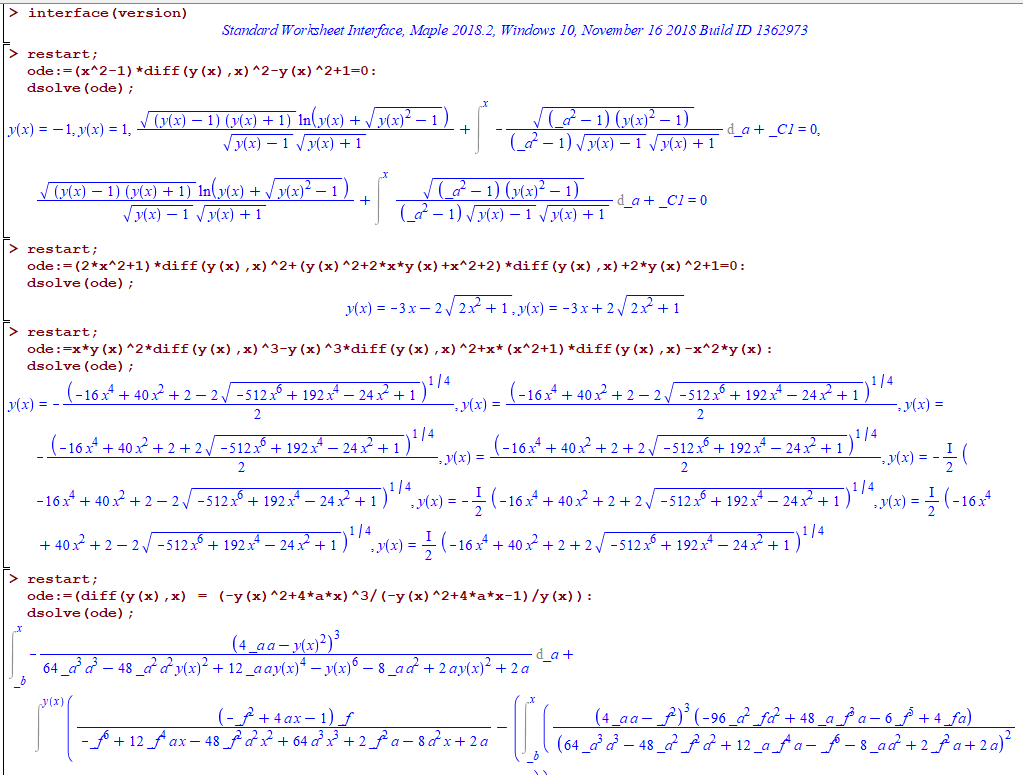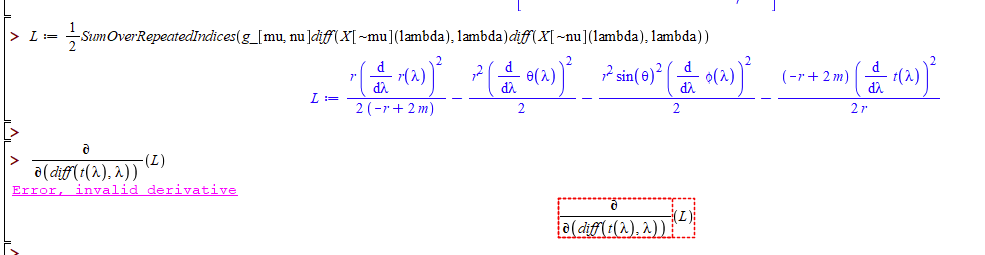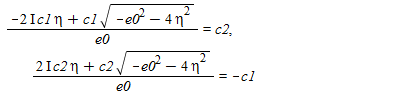## How do I put a line all to run from a particular p...

How can I put a line to run from a single point on the x-axis to the top. Like a like that run at x = 5 axis to the top.

## How to choose which line and which color Plot on t...

Du plot two lines but overlapped

how to set color of each line and choose each line plot on top of layer to confirm overlapped?

## why these ODE's hang or return unsolved in Maple 2...

When I tried these ODE's in Maple 2019, and Maple 2018, they are worked (i.e. gave solutions). Now these ODE's either hang in 2020 or return unsolved. What could have caused this? On Maple 2020, under windows 10:

```restart;
ode:=(x^2-1)*diff(y(x),x)^2-y(x)^2+1=0:
dsolve(ode); #hangs

restart;
ode:=(2*x^2+1)*diff(y(x),x)^2+(y(x)^2+2*x*y(x)+x^2+2)*diff(y(x),x)+2*y(x)^2+1=0:
dsolve(ode); # return unsolved

restart;
ode:=x*y(x)^2*diff(y(x),x)^3-y(x)^3*diff(y(x),x)^2+x*(x^2+1)*diff(y(x),x)-x^2*y(x):
dsolve(ode); # return unsolved

restart;
ode:=(diff(y(x),x) = (-y(x)^2+4*a*x)^3/(-y(x)^2+4*a*x-1)/y(x)):
dsolve(ode); #hangs
```

I noticed that in Maple 2019.2 this problem is also there. But not in Maple 2019.  So something changed from after Maple 2019.

Here is worksheet on Maple 2018 that shows these worked there. But no longer work on Maple 2020. There might be more like these but these the ones I found so far.issue.mw

## Input in percent (%) or ­‰...

Is it possible to define variables with "unit" % or ‰ in Maple?

`c:=20%`

does not work, it apparently gives you 20 multiplied with the result of the last calculation.

## A different kind of differentiation...

Hi folks!

Is there any way to make this kind of derivative?If necessary, I can provide the original file here.

Thanks in advance for any help!

## Working with vectors...

If I understand how to use vectors in Maple, I need to give the number of elements.  Is this correct?  I am working with vectors that I do not know the number of elements.  I can use a large number that will be greater than the required number of elements, but this does not seem to be a good solution.  The number of elements is 2000 for about 10 different vectors.  What is the best method to set up the victors for this application?

## Fractional Reduced Differential Transform Method...

Can anyone help me to frame the equations in Fractional Reduced Differential Transform Method

system of nonlinear ordinary diﬀerential equations
ds/ dt = b−γ s(t)− (δ s(t)(i(t) + βa(t)) /N − ε s(t) m(t)
de/ dt = δ (s(t)(i(t) + βa(t))/ N + ε s(t) m(t) − (1−ϑ) θ e(t) − ϑ α e(t) − γ e(t)
di/ dt = (1−ϑ) θ e(t) − (ρ + γ) i(t)
da/ dt = ϑ α e(t) − (σ + γ) a(t)
dr /dt = ρ i(t) + σ a(t) − γ r(t)
dm /dt = τ i(t) + κ a(t) − ω m(t)

## How can I select 2 variables from 3 to obtain a co...

Good day to all.

I have a function, z(x,y), that I am interested in exploring. The contour plot returns the z-contours on the x-y grid (see attached).

Can anyone suggest a way in which I can now assign z to the vertical axis (instead of y), x to the horizontal axis, and plot the contours for various y-values?

Contour_Plot_MaplePrimes.mw

## 2D Polar Coordinate Plot...

How can I  get the "phase portrait including vector fields" and several trajectories of the following dynamical system in the polar coordinates (Note that the system is also in polar coordinate)??## How can we remove c1 from the expression of c2 and...

(-(2*I)*c1*eta+c1*sqrt(-e0^2-4*eta^2))/e0 = c2, ((2*I)*c2*eta+c2*sqrt(-e0^2-4*eta^2))/e0 = -c1How can we remove c1 from the expression of c2 and similarly c2 from c1?

## SolidOfRevolution ...

Hi

I try to visualize and animate the hollow volume between two functions, but I do not have an optimal rendering

Ideas? Thanks

## Why are the Gauss-Bonnet figures incorrect in my w...

The worksheet below displays dual cones inside the unit sphere, but the Gauss-Bonnet formula (area of upper cone's spherical cap plus length of dual cone's intersection with unit sphere) does not equal 2*Pi.

Dual_cones.mw

## How do I turn a system of parametric equations int...

In Maple 2020, I clicked on "Math Apps" and then clicked on "Algebra & Geometry", followed by "Boy's Surface".

It is a set of 3 parametric equations in terms of {u,v}. I would like to eliminate u and v and turn this into a single "error" function in terms of (x,y,z) such that anywhere this function is zero, the original set of equations are satisfied for some value of {u,v}. (Ideally, the values of u and v could be determined as well, but that would be a bonus.)

I tried this:

alpha = 1;
u in [-Pi/2, Pi/2];
v in [0, Pi];
eq1 := x(u, v) = (sqrt(2)*cos(v)^2*cos(2*u) + cos(u)*sin(2*v))/(2 - alpha*sqrt(2)*sin(3*u)*sin(2*v));
eq2 := y(u, v) = (sqrt(2)*cos(v)^2*sin(2*u) - sin(u)*sin(2*v))/(2 - alpha*sqrt(2)*sin(3*u)*sin(2*v));
eq3 := z(u, v) = 3*cos(v)^2/(2 - alpha*sqrt(2)*sin(3*u)*sin(2*v));
eliminate({eq1, eq2, eq3}, {u, v});

but got no response and no error message.

Additionally, I tried explaining that I want a function f such that f(x,y,z)=0, but again got no response and no error message:

eq4 := f(x, y, z) = 0;
solve({eq1, eq2, eq3, eq4}, f);

Any ideas what I'm doing wrong?

Thank you!

-- Glenn

## Finding the intersection point...

Hellow everyone,

Consider the following diffrential equation:

(dA(x)/dx )^2+V(A)=0,
1- How to plot dA/dx vs A?

2- How to find the coordinate of intersection point of f(x)=e-b*exp(x)-c*(1-2*x/c)^(1/2) with df/dx? e,b,c are constants.

## Can anyone tell me what is causing this error and ...

Hi everyone! I was writing this code for an interactive component, when I stumbled into a problem that I couldn't solve...

Here is the code.

use DocumentTools in
if type(Do(%NIniziale), posint) then
if Do(%NIterazioni)<=100 then
if type(Do(%NIterazioni), posint) then
Do(%E="Valid Values!");
Even:=Do(%Pari);
Odd:=Do(%Dispari);
Iterazioni:=proc (N, i)
local m, n;
n:=N;
for m from 1 to i do
if type(n, even) = true then
n := Even;
else
n := Odd;
end if;
end do;
return (SetCellFormula("Risultati", i, 1, eval(n)));
end proc;
ix:=Do(eval(%NIterazioni));
Nx:=Do(eval(%NIniziale));
seq(Iterazioni(Nx,y),y=1..eval(ix));
if Do(%NIterazioni)<100 then
seq(SetCellFormula("Risultati", x, 1, "//"), x=1+ix..100);
end if;
else
Do(%E="Inavalid Values");
seq(SetCellFormula("Risultati", x, 1, "ERROR"), x=1..100);
end if;
else
Do(%E="Invalid Values");
seq(SetCellFormula("Risultati", x, 1, "ERROR"), x=1..100);
end if;
else
Do(%E="Invalid Values");
seq(SetCellFormula("Risultati", x, 1, "ERROR"), x=1..100);
end if;
end use;

Now this are what the names stands for:

%Pari is a math conainer in which there is a function of n that is to be used when n is even;

%Dispari is a math conainer in which there is a function of n that is to be used when n is odd;

"Risultati" is the name of a spreadsheet on which the results will be displayed;

%NIterazioni is a math conainer in which there is a posint that indicates how many times the procedure is to be iterated;

%NIniziale is a math conainer in which there is the starting number (another posint) to which on which the procedure will operate;

%E is a text area that will signal the validity of the input.

What I wanted to do was a procedure that reiterated two function on a given number a given amount of times and returned the result of every passage, however, when computing, it returns the function in n and not the final numbers.